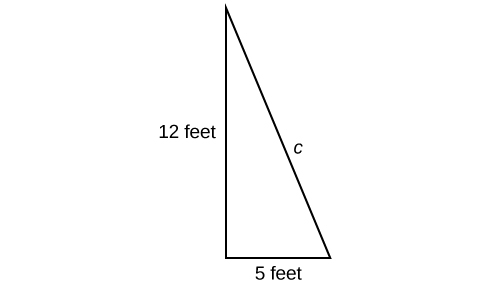## Putting it Together: Roots and Rational ExponentsCat heaven

At the beginning of this lesson, we left Joan and her brother who were planning to make a ramp for Joan’s cat Hobbes from her window. She used the Pythagorean Theorem to get this far, but was left with an equation she did not know how to solve:

$\begin{array}{ccc}\hfill {a}^{2}+{b}^{2}& =& {c}^{2}\hfill \\ \hfill {5}^{2}+{12}^{2}& =& {c}^{2}\hfill \\ \hfill 169& =& {c}^{2}\hfill \end{array}$

The goal was to find the unknown length in the following triangle, where c represents the length of the cat ladder.Joan’s brother, Jacob, suggested that she use a square root to find the solution to her equation. Joan remembered that roots and exponents are related – one “undoes” the other. She tried it out in the following way:

$\begin{array}{ccc}169 ={c}^{2}\\\sqrt{169}=\sqrt{c^2}\\13=c\end{array}$

Hooray! Joan has the length she needs to create her cat’s ladder. Hopefully she will be able to get some sleep now.